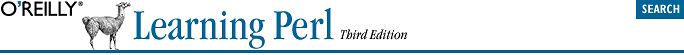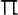home | O'Reilly's CD bookshelfs | FreeBSD | Linux | Cisco | Cisco Exam## 2.13. Exercises

See Section A.1, "Answers to Chapter 2 Exercises " for answers to the following exercises:

1.  Write a program that computes the circumference of a circle with a radius of 12.5. Circumference is 2times the radius (approximately 2 times 3.141592654). The answer you get should be about 78.5.

2.  Modify the program from the previous exercise to prompt for and accept a radius from the person running the program. So, if the user enters 12.5 for the radius, she should get the same number as in the previous exercise.

3.  Modify the program from the previous exercise so that, if the user enters a number less than zero, the reported circumference will be zero, rather than negative.

4.  Write a program that prompts for and reads two numbers (on separate lines of input) and prints out the product of the two numbers multiplied together.

5.  Write a program that prompts for and reads a string and a number (on separate lines of input) and prints out the string the number of times indicated by the number on separate lines. (Hint: Use the "x" operator.) If the user enters "fred" and "3," the output should be three lines, each saying "fred". If the user enters "fred" and "299792," there may be a lot of output.